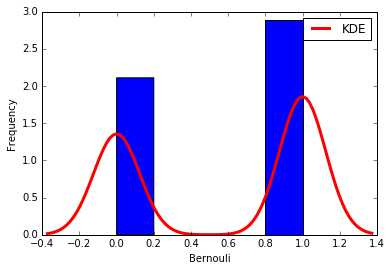# Data Science different topic's explanation -- Part-10 -- Discrete distributions

This is data science different topic explanation conversation series.
If you are not following below mentioned are the links for each part.

Part-1:

Part-2:

Part-3:

Part-4:

Part-5:

Part-6:

Part-7:

Part-8:

Part-9:

# Data Science different topic’s explanation – Part-10 – Discrete distributions

## Bernoulli distribution (binomial distribution)

``````from scipy.stats import bernoulli
import seaborn as sb

def bernoulli_dist(): -> None:
data_bern = bernoulli.rvs(size=1000,p=0.6)
ax = sb.distplot(
data_bern,
kde=True,
color='b',
hist_kws={'alpha':1},
kde_kws={'color': 'r', 'lw': 3, 'label': 'KDE'})
ax.set(xlabel='Bernouli', ylabel='Frequency')

bernoulli_dist()
``````Not all phenomena are measured on a quantitative scale of type 1,2,3 … 100500… Not always a phenomenon can take on an infinite or a large number of different states. For instance, a person’s sex can be either a man or a woman. The shooter either hits the target or missed. You can vote either “for” or “against”, etc. Other words reflect the state of an alternative feature (the event did not come).

Continuing the left out content here of above post.

The upcoming event (positive outcome) is also called “success”. Such phenomena can also be massive and random. Therefore, they can be measured and make statistically valid conclusions.

Experiment with such data are called the Bernoulli scheme, in honor of the famous Swiss mathematician, who found that with a large number of tests, the ratio of positive outcomes to the total number of tests converges to the probability of the occurrence of this event.

Continuing the missed out content here.

• `n` – the number of experiments in the series;
• `x` – a random variable (the number of occurrences of the event);
• `Px` – the probability that event happens exactly `x` times;
• `q = 1 - p` (the probability that the event does not appear in the test)